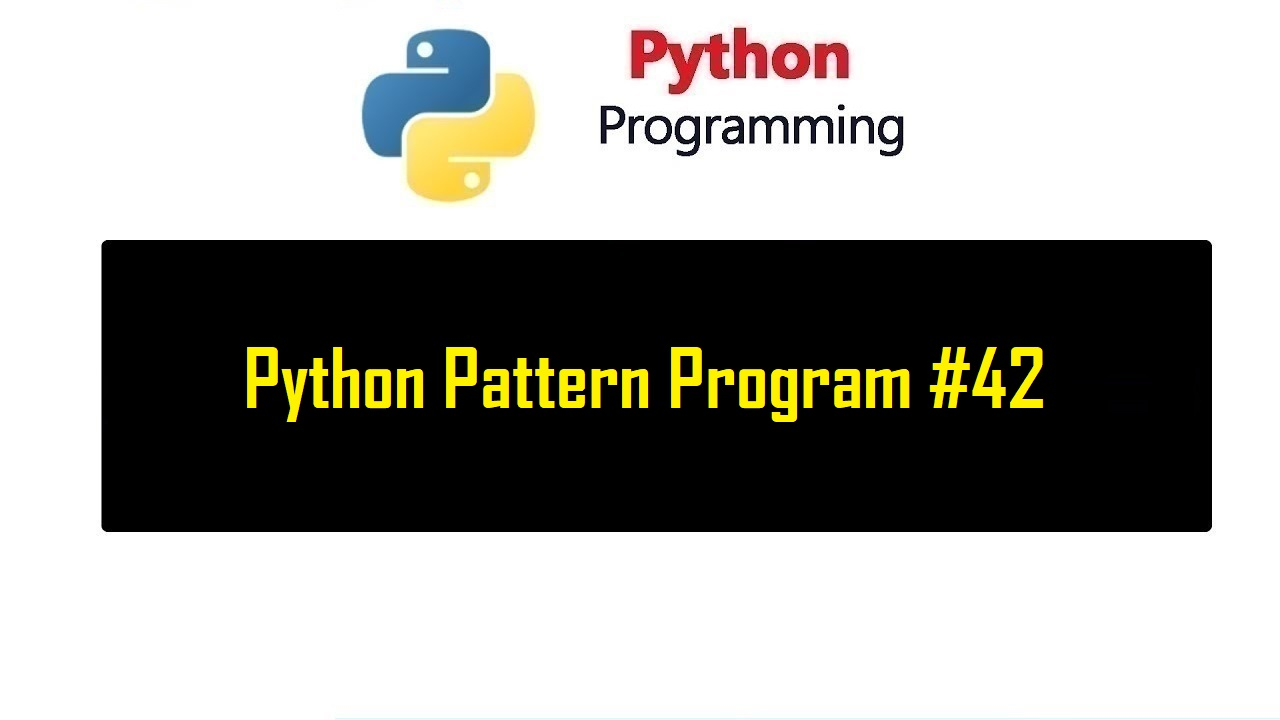# Python Pattern Program #42

0

##Python Pattern Program #42

### Source Code:

n=int(input("Enter the number of rows: "))
for i in range(1,n+1):
print(" "*(n-i),end="")
for j in range(1,i):
print(i-j,end=" ")
for k in range(0,i):
print(k,end=" ")
print()

Tags